# RD Sharma Solutions for Class 10 Chapter 11 Constructions Exercise 11.2

Students can understand the methods of constructing a triangle similar to a given triangle in this exercise. The RD Sharma Solutions Class 10 has all the answers prepared by experts at BYJU’s for clearing doubts and giving the correct methodologies to solve a problem. Students can be greatly benefited from this resource. The RD Sharma Solutions for Class 10 Maths Chapter 11 Constructions Exercise 11.2 PDF is also provided below for students to help them with the right steps in solving questions.

## RD Sharma Solutions for Class 10 Chapter 11 Constructions Exercise 11.2 Download PDF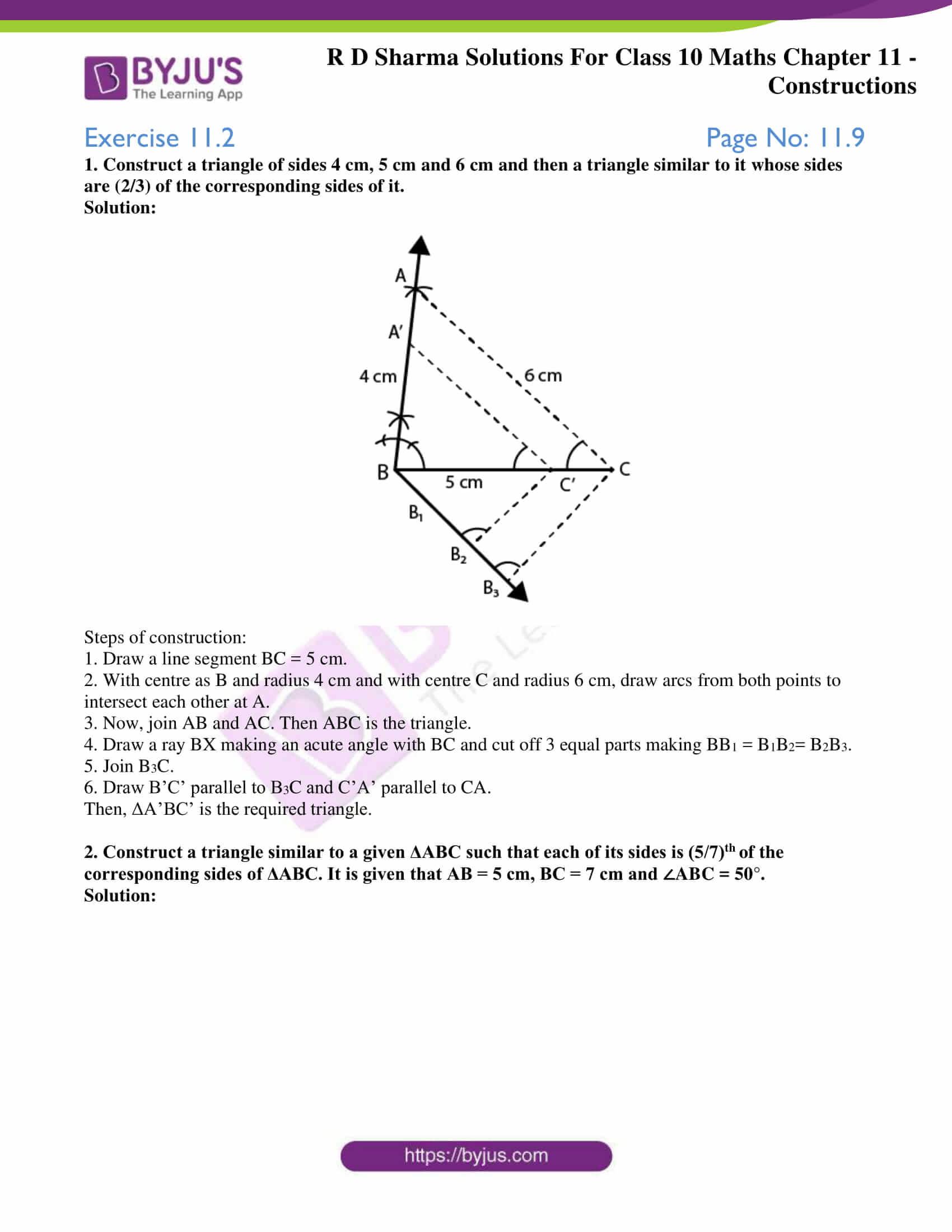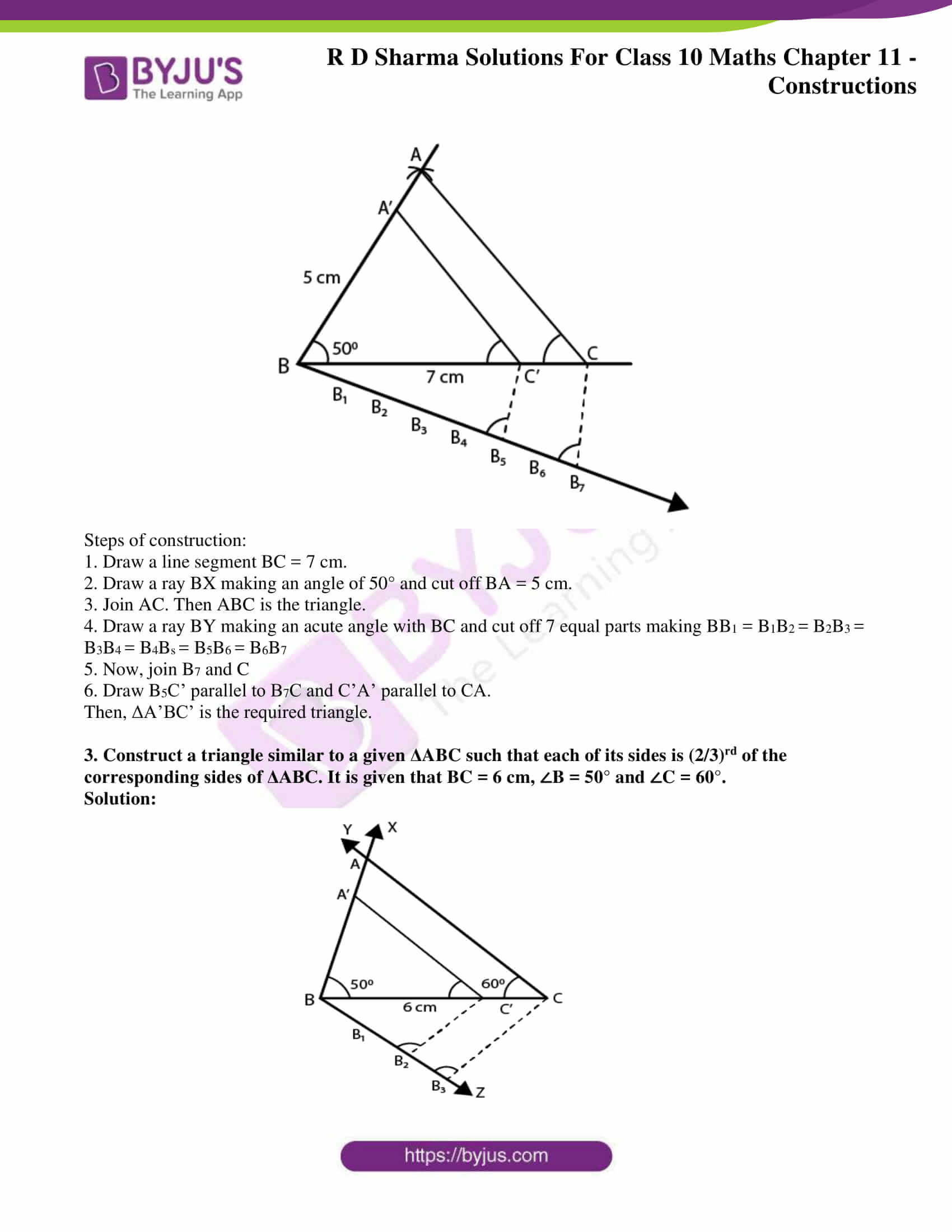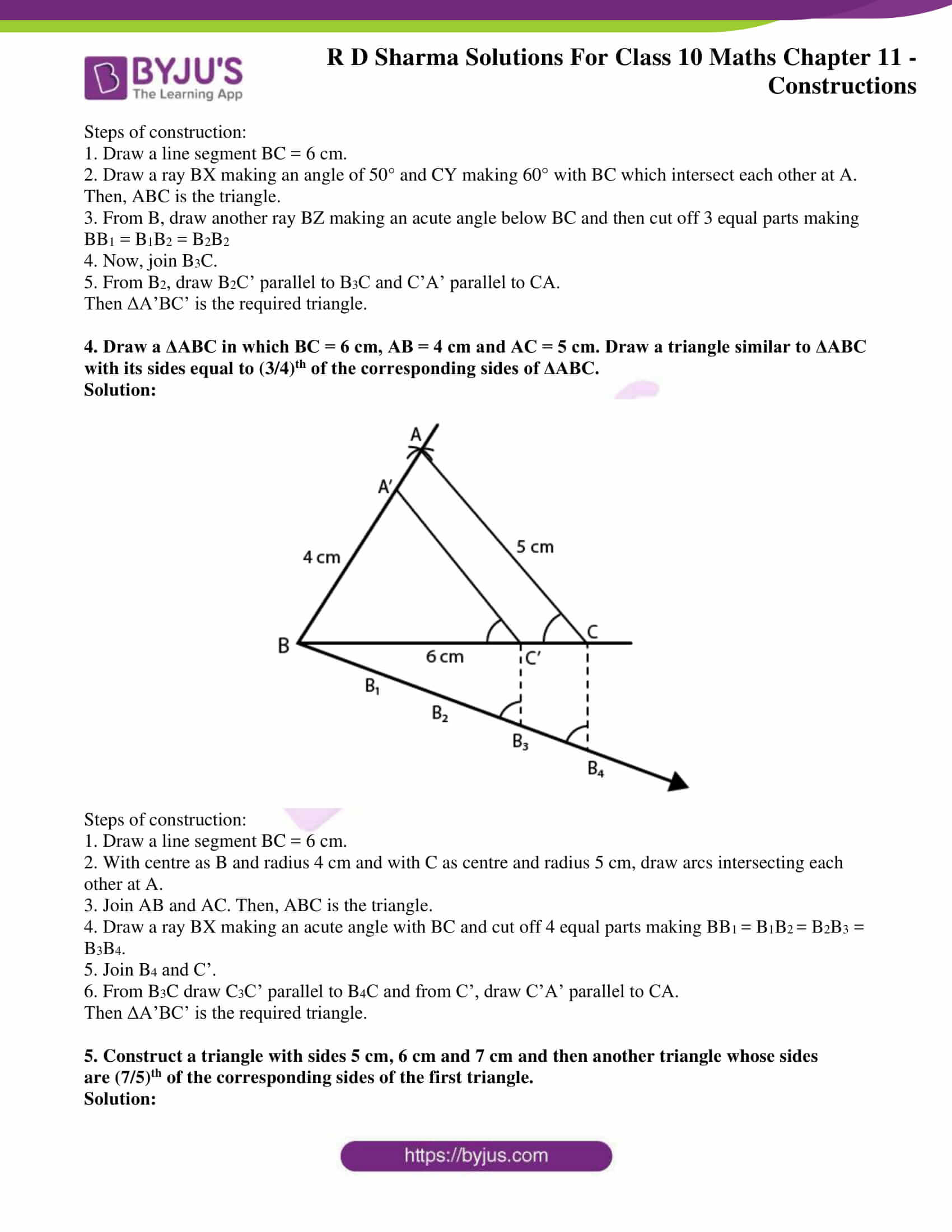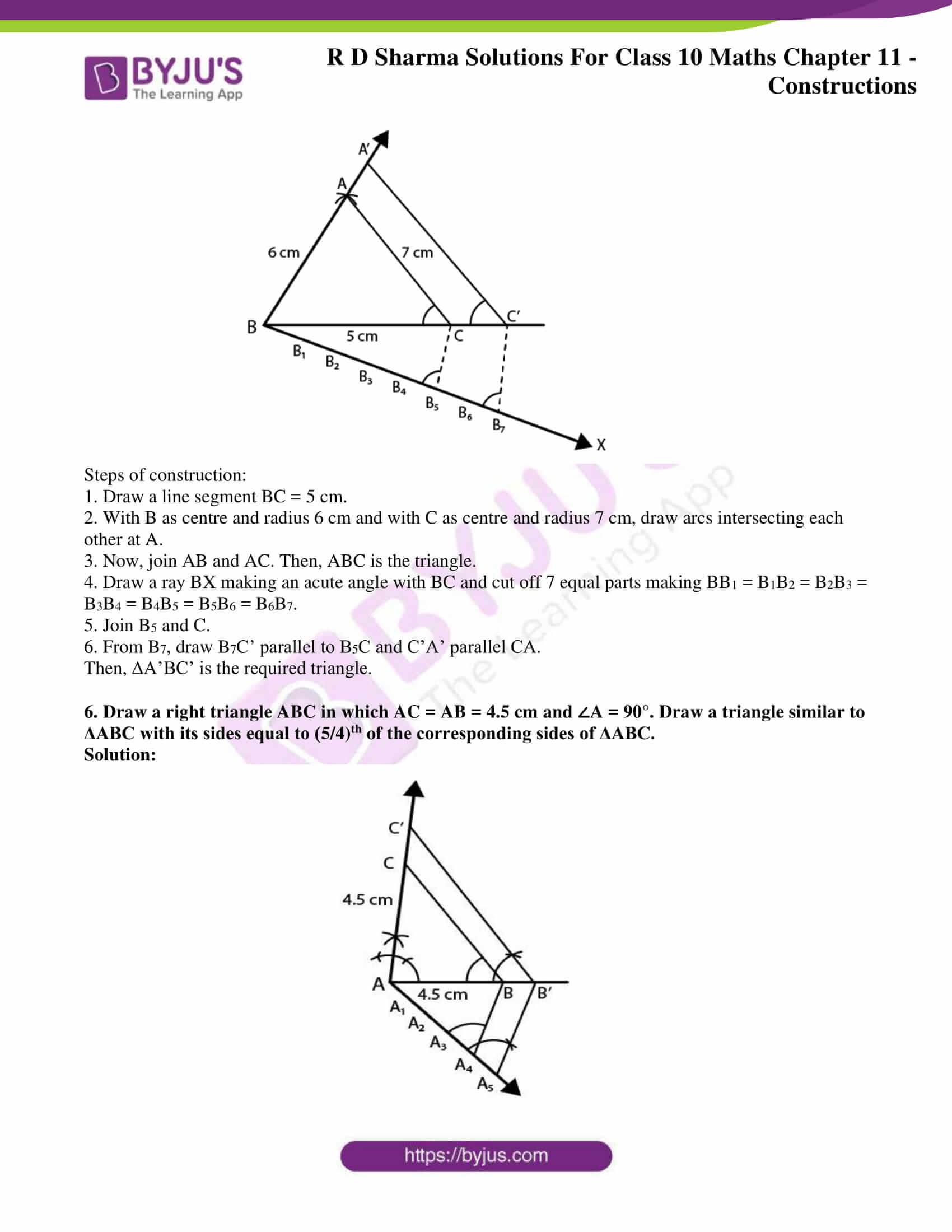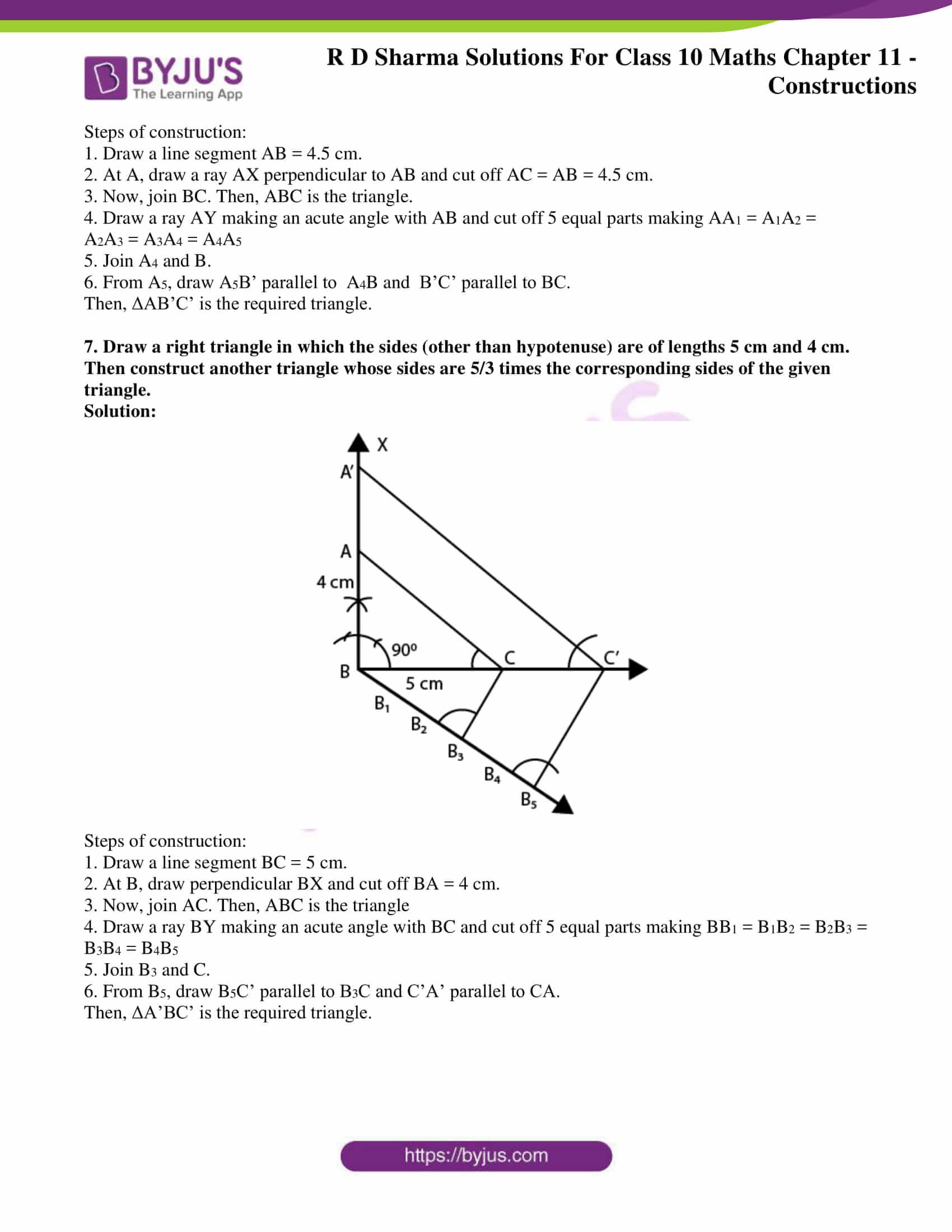### Access RD Sharma Solutions for Class 10 Chapter 11 Constructions Exercise 11.2

1. Construct a triangle of sides 4 cm, 5 cm and 6 cm and then a triangle similar to it whose sides are (2/3) of the corresponding sides of it.
Solution: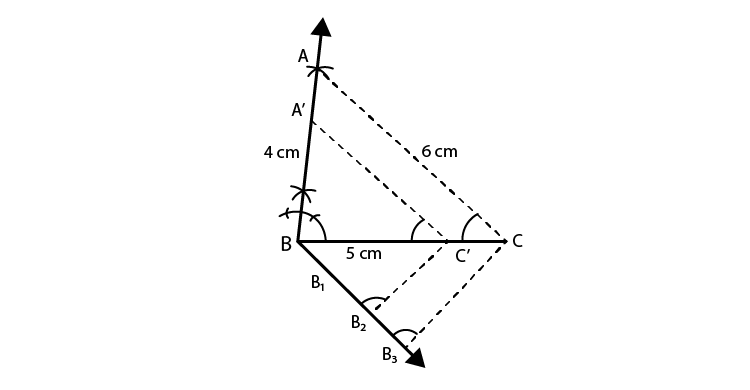Steps of construction:

1. Draw a line segment BC = 5 cm.

2. With centre as B and radius 4 cm and with centre C and radius 6 cm, draw arcs from both points to intersect each other at A.

3. Now, join AB and AC. Then ABC is the triangle.

4. Draw a ray BX making an acute angle with BC and cut off 3 equal parts making BB1 = B1B2= B2B3.

5. Join B3C.

6. Draw B’C’ parallel to B3C and C’A’ parallel to CA.

Then, ΔA’BC’ is the required triangle.

2. Construct a triangle similar to a given ΔABC such that each of its sides is (5/7)th of the corresponding sides of ΔABC. It is given that AB = 5 cm, BC = 7 cm and ∠ABC = 50°.
Solution: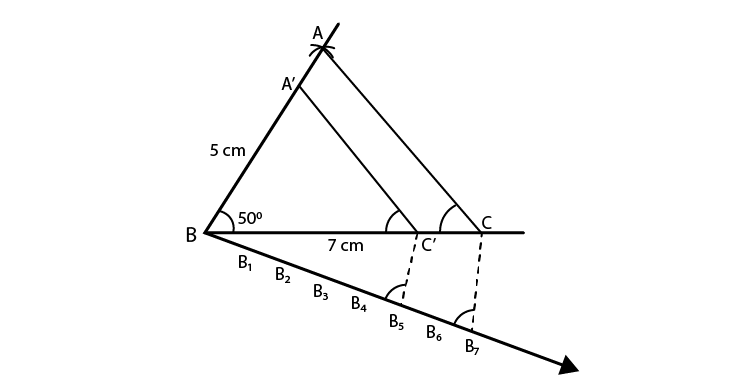Steps of construction:

1. Draw a line segment BC = 7 cm.

2. Draw a ray BX making an angle of 50° and cut off BA = 5 cm.

3. Join AC. Then ABC is the triangle.

4. Draw a ray BY making an acute angle with BC and cut off 7 equal parts making BB1 = B1B2 = B2B3 = B3B4 = B4Bs = B5B6 = B6B7

5. Now, join B7 and C

6. Draw B5C’ parallel to B7C and C’A’ parallel to CA.

Then, ΔA’BC’ is the required triangle.

3. Construct a triangle similar to a given ΔABC such that each of its sides is (2/3)rd of the corresponding sides of ΔABC. It is given that BC = 6 cm, ∠B = 50° and ∠C = 60°.
Solution: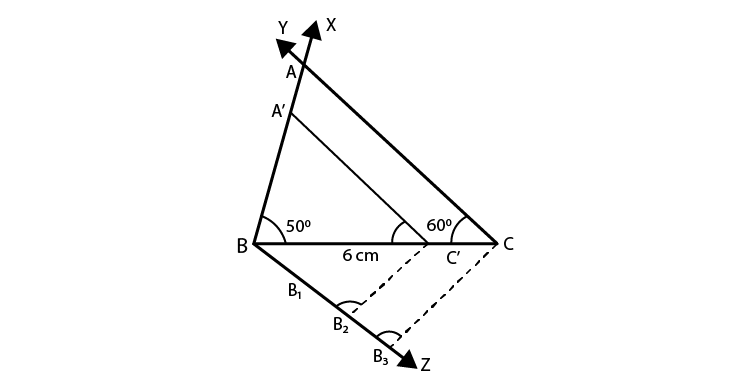Steps of construction:

1. Draw a line segment BC = 6 cm.

2. Draw a ray BX making an angle of 50° and CY making 60° with BC which intersect each other at A. Then, ABC is the triangle.

3. From B, draw another ray BZ making an acute angle below BC and then cut off 3 equal parts making BB1 = B1B2 = B2B2

4. Now, join B3C.

5. From B2, draw B2C’ parallel to B3C and C’A’ parallel to CA.

Then ΔA’BC’ is the required triangle.

4. Draw a ΔABC in which BC = 6 cm, AB = 4 cm and AC = 5 cm. Draw a triangle similar to ΔABC with its sides equal to (3/4)th of the corresponding sides of ΔABC.
Solution: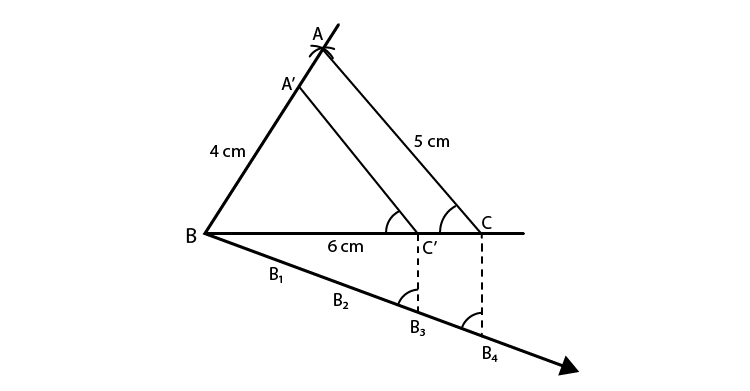Steps of construction:

1. Draw a line segment BC = 6 cm.

2. With centre as B and radius 4 cm and with C as centre and radius 5 cm, draw arcs intersecting each other at A.

3. Join AB and AC. Then, ABC is the triangle.

4. Draw a ray BX making an acute angle with BC and cut off 4 equal parts making BB1 = B1B= B2B3 = B3B4.

5. Join B4 and C’.

6. From B3C draw C3C’ parallel to B4C and from C’, draw C’A’ parallel to CA.

Then ΔA’BC’ is the required triangle.

5. Construct a triangle with sides 5 cm, 6 cm and 7 cm and then another triangle whose sides are (7/5)th of the corresponding sides of the first triangle.
Solution: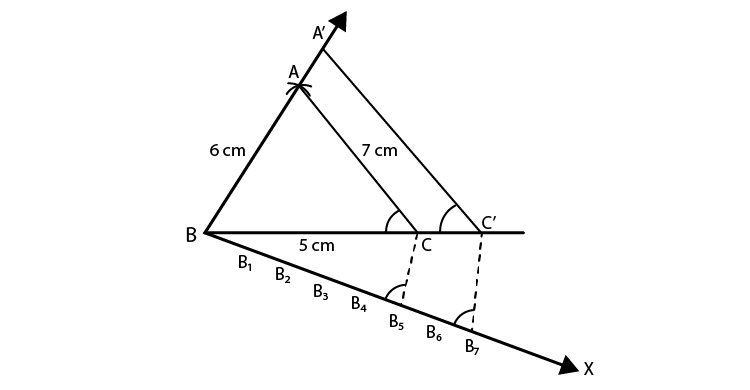Steps of construction:

1. Draw a line segment BC = 5 cm.

2. With B as centre and radius 6 cm and with C as centre and radius 7 cm, draw arcs intersecting each other at A.

3. Now, join AB and AC. Then, ABC is the triangle.

4. Draw a ray BX making an acute angle with BC and cut off 7 equal parts making BB1 = B1B2 = B2B3 = B3B4 = B4B5 = B5B6 = B6B7.

5. Join B5 and C.

6. From B7, draw B7C’ parallel to B5C and C’A’ parallel CA.

Then, ΔA’BC’ is the required triangle.

6. Draw a right triangle ABC in which AC = AB = 4.5 cm and ∠A = 90°. Draw a triangle similar to ΔABC with its sides equal to (5/4)th of the corresponding sides of ΔABC.
Solution: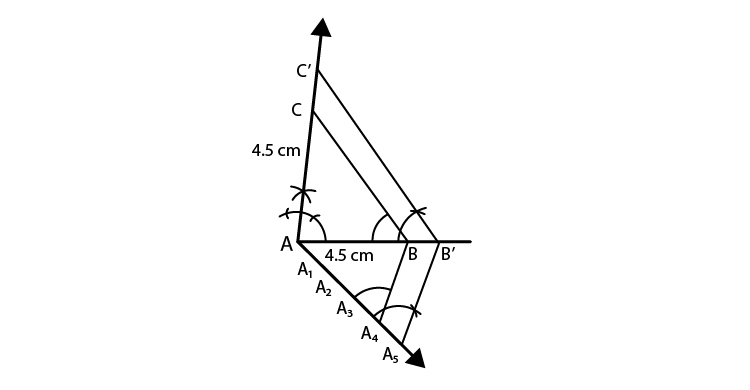Steps of construction:

1. Draw a line segment AB = 4.5 cm.

2. At A, draw a ray AX perpendicular to AB and cut off AC = AB = 4.5 cm.

3. Now, join BC. Then, ABC is the triangle.

4. Draw a ray AY making an acute angle with AB and cut off 5 equal parts making AA1 = A1A2 = A2A3 = A3A4 = A4A5

5. Join A4 and B.

6. From A5, draw A5B’ parallel to  A4B and  B’C’ parallel to BC.

Then, ΔAB’C’ is the required triangle.

7. Draw a right triangle in which the sides (other than hypotenuse) are of lengths 5 cm and 4 cm. Then construct another triangle whose sides are 5/3 times the corresponding sides of the given triangle.
Solution: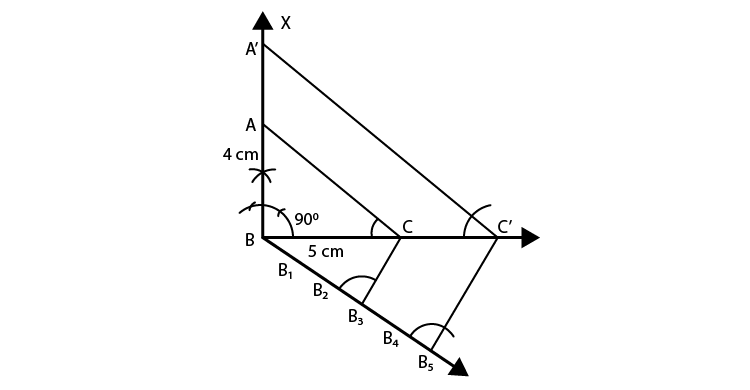Steps of construction:

1. Draw a line segment BC = 5 cm.

2. At B, draw perpendicular BX and cut off BA = 4 cm.

3. Now, join AC. Then, ABC is the triangle

4. Draw a ray BY making an acute angle with BC and cut off 5 equal parts making BB1 = B1B2 = B2B3 = B3B4 = B4B5

5. Join B3 and C.

6. From B5, draw B5C’ parallel to B3C and C’A’ parallel to CA.

Then, ΔA’BC’ is the required triangle.